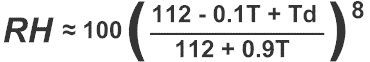Temperature Conversion
Enter a number then click on the appropriate number to see the result.
Fahrenheit (F)

 Celsius (C)

Wind Chill/Temperature Index Calculation in degrees Fahrenheit

Enter in the air temperature ( Tair ) in degrees Fahrenheit and wind speed in mile per hour ( mph ), then click on the Calculate WC to compute the windchill ( WC ).

Tair F mph

Wind Chill/Temperature Index Calculation in degrees Celsius

Enter in the air temperature ( Tair ) in degrees Celsius and wind speed in miles per hour, then click on the Calculate WC to compute the windchill ( WC ).

Tair C mph
Relative Humidity Calculation using degrees Fahrenheit

Enter in the air temperature ( Tair ) and dew point temperature ( Tdp) in degrees Fahrenheit ( °CF ) then click on the Calculate RH to compute the relative humidity ( RH ).
Tair in °F Tdp in °F RH
Tair
Tdp

Relative Humidity Calculation using degrees Celsius

Enter in the air temperature ( Tair ) and dew point temperature ( Tdp) in degrees Celsius ( °CF ) then click on the Calculate RH to compute the relative humidity ( RH ).
Tair in °C Tdp in °C RH
Tair
Tdp
Heat Index Calculation

Valid entries are, air temperatures greater than 80 °F ( 27 °C ), dew point temperatures greater than 65 °F ( 12 °C ), and relative humidities higher than 40 percent.

in Celsius

Enter in the air temperature ( Tair ) and the dew point temperature ( Tdp ) in degrees Celsius, then click on the Calculate HI to compute the heat index ( HI).
Tair in °C Tdp in °C RH =

in Fahrenheit

Enter in the air temperature ( Tair ) the dew point temperature ( Tdp ) in degrees Fahrenheit, then click on the Calculate HI to compute the heat index ( HI).

Tair in °F Tdp in °F RH =

in Celsius

Enter in the air temperature ( Tair ) in degrees Celsius and relative humidity ( RH ) in percent ( without the % sign ), then click on the Calculate HI to compute the heat index ( HI ).

Tair in °C RH Tdp =

in Fahrenheit

Enter in the air temperature ( Tair ) in degrees Fahrenheit and relative humidity ( RH ) in percent ( without the % sign ), then click on the Calculate HI to compute the heat index ( HI ).
Tair in °F RH Tdp =